Albert Einstein: Relativity
Appendix

## Simple Derivation of the Lorentz Transformation(Supplementary to Section 11)

For the relative orientation of the co-ordinate systems indicated in Fig. 2, the x-axes of both systems pernumently coincide. In the present case we can divide the problem into parts by considering first only events which are localised on the x-axis. Any such event is represented with respect to the co-ordinate system K by the abscissa x and the time t, and with respect to the system K1 by the abscissa x' and the time t'. We require to find x' and t' when x and t are given.

A light-signal, which is proceeding along the positive axis of x, is transmitted according to the equation

x = ct

or

x - ct = 0     .     .     .    (1).

Since the same light-signal has to be transmitted relative to K1 with the velocity c, the propagation relative to the system K1 will be represented by the analogous formula

x' - ct' = O     .     .     .    (2)

Those space-time points (events) which satisfy (x) must also satisfy (2). Obviously this will be the case when the relation

(x' - ct') = λ (x - ct)     .     .     .    (3).

is fulfilled in general, where λ indicates a constant ; for, according to (3), the disappearance of (x - ct) involves the disappearance of (x' - ct').

If we apply quite similar considerations to light rays which are being transmitted along the negative x-axis, we obtain the condition

(x' + ct') = µ(x + ct)    .     .     .    (4).

By adding (or subtracting) equations (3) and (4), and introducing for convenience the constants a and b in place of the constants λ and µ, where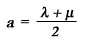and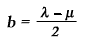we obtain the equations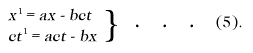We should thus have the solution of our problem, if the constants a and b were known. These result from the following discussion.

For the origin of K1 we have permanently x' = 0, and hence according to the first of the equations (5)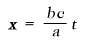If we call v the velocity with which the origin of K1 is moving relative to K, we then have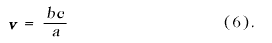The same value v can be obtained from equations (5), if we calculate the velocity of another point of K1 relative to K, or the velocity (directed towards the negative x-axis) of a point of K with respect to K'. In short, we can designate v as the relative velocity of the two systems.

Furthermore, the principle of relativity teaches us that, as judged from K, the length of a unit measuring-rod which is at rest with reference to K1 must be exactly the same as the length, as judged from K', of a unit measuring-rod which is at rest relative to K. In order to see how the points of the x-axis appear as viewed from K, we only require to take a " snapshot " of K1 from K; this means that we have to insert a particular value of t (time of K), e.g. t = 0. For this value of t we then obtain from the first of the equations (5)

x' = ax

Two points of the x'-axis which are separated by the distance Δx' = I when measured in the K1 system are thus separated in our instantaneous photograph by the distance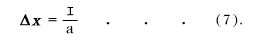But if the snapshot be taken from K'(t' = 0), and if we eliminate t from the equations (5), taking into account the expression (6), we obtain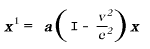From this we conclude that two points on the x-axis separated by the distance I (relative to K) will be represented on our snapshot by the distance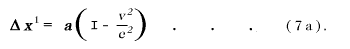But from what has been said, the two snapshots must be identical; hence Δx in (7) must be equal to Δx' in (7a), so that we obtain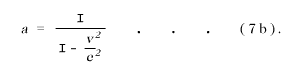The equations (6) and (7b) determine the constants a and b. By inserting the values of these constants in (5), we obtain the first and the fourth of the equations given in Section 11.Thus we have obtained the Lorentz transformation for events on the x-axis. It satisfies the condition

x'2 - c2t'2 = x2 - c2t2    .     .     .    (8a).

The extension of this result, to include events which take place outside the x-axis, is obtained by retaining equations (8) and supplementing them by the relations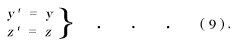In this way we satisfy the postulate of the constancy of the velocity of light in vacuo for rays of light of arbitrary direction, both for the system K and for the system K'. This may be shown in the following manner.

We suppose a light-signal sent out from the origin of K at the time t = 0. It will be propagated according to the equation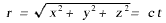or, if we square this equation, according to the equation

x2 + y2 + z2 = c2t2 = 0    .     .     .    (10).

It is required by the law of propagation of light, in conjunction with the postulate of relativity, that the transmission of the signal in question should take place — as judged from K1 — in accordance with the corresponding formula

r' = ct'

or,

x'2 + y'2 + z'2 - c2t'2 = 0    .     .     .    (10a).

In order that equation (10a) may be a consequence of equation (10), we must have

x'2 + y'2 + z'2 - c2t'2 = σ (x2 + y2 + z2 - c2t2)       (11).

Since equation (8a) must hold for points on the x-axis, we thus have σ = I. It is easily seen that the Lorentz transformation really satisfies equation (11) for σ = I; for (11) is a consequence of (8a) and (9), and hence also of (8) and (9). We have thus derived the Lorentz transformation.

The Lorentz transformation represented by (8) and (9) still requires to be generalised. Obviously it is immaterial whether the axes of K1 be chosen so that they are spatially parallel to those of K. It is also not essential that the velocity of translation of K1 with respect to K should be in the direction of the x-axis. A simple consideration shows that we are able to construct the Lorentz transformation in this general sense from two kinds of transformations, viz. from Lorentz transformations in the special sense and from purely spatial transformations. which corresponds to the replacement of the rectangular co-ordinate system by a new system with its axes pointing in other directions.

Mathematically, we can characterise the generalised Lorentz transformation thus :

It expresses x', y', x', t', in terms of linear homogeneous functions of x, y, x, t, of such a kind that the relation

x'2 + y'2 + z'2 - c2t'2 = x2 + y2 + z2 - c2t2       (11a).

is satisficd identically. That is to say: If we substitute their expressions in x, y, x, t, in place of x', y', x', t', on the left-hand side, then the left-hand side of (11a) agrees with the right-hand side.